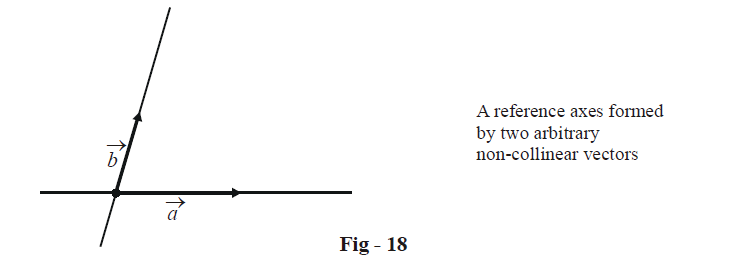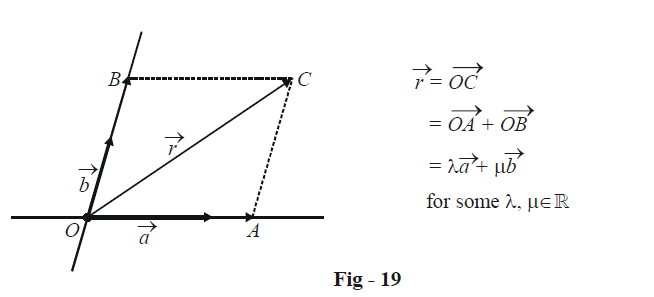# Basis Of A Two Dimensional Plane

THE BASIS OF A VECTOR SPACE

Consider a two-dimensional plane, and any two arbitrary non-collinear vectors $$\vec a\,\,{\text{and}}\,\,\vec b$$  in this plane. We make the two vectors co-initial and use their supports as our reference axes:Observe carefully that any vector $$\vec r$$ in the plane can be represented in terms of  $$\vec a\,\,{\text{and}}\,\,\vec b$$ . We find the components of  $$\vec r$$ along the directions of $$\vec a\,\,{\text{and}}\,\,\vec b$$; those components must be some scalar multiples of $$\vec a\,\,{\text{and}}\,\,\vec b$$ .Thus, any vector $$\vec r$$  in the plane can be written as

$\vec r = \lambda \vec a + \mu \vec b\;\qquad\qquad\qquad for{\text{ }}some\;\lambda ,\mu \in \mathbb{R}{\text{ }}\quad\qquad\qquad...{\text{ }}\left( 1 \right)$

i.e, any vector $$\vec r$$  in the plane can be expressed as a linear combination of  $$\vec a\,\,{\text{and}}\,\,\vec b$$.

We state this fact in mathematical terms as follows: the vectors $$\vec a\,\,{\text{and}}\,\,\vec b$$ form a basis of our vector space (which is a plane in this case). The term “basis” means that using only $$\vec a\,\,{\text{and}}\,\,\vec b$$ , we can construct any vector lying in the plane of  $$\vec a\,\,{\text{and}}\,\,\vec b$$ .

Note that there’s nothing special about  $$\vec a\,\,{\text{and}}\,\,\vec b$$ ; any two non-collinear vectors can form a basis for the plane.

You must be very clear on the point that two collinear vectors cannot form the basis for a plane while any two non-collinear vectors can. Understanding this fact is very crucial to later discussions.

Try proving this: let $$\vec a\,\,{\text{and}}\,\,\vec b$$  form the basis of a plane. For any vector $$\vec r$$ in the plane of $$\vec a\,\,{\text{and}}\,\,\vec b$$ , we can find scalars $$\lambda ,\mu \in \mathbb{R}$$ such that

$\vec r = \lambda \vec a + \mu \vec b$

Prove that this representation is unique.

The basic principle that we’ve learnt in this discussion can be expressed in a very useful way as follows:

 Three vectors are coplanar if and only if one of them can be expressed as a linear combination of the other two. i.e., three vectors $$\vec a,\,\,\vec b,\,\,\vec c$$ are coplanar if there exist scalars  $${l_1},{l_2} \in \mathbb{R}$$  such that                     $\vec a = {l_1}\vec b + {l_2}\vec c$ We can write this as                                    (1)$$\vec a + \left( { - {l_1}} \right)\vec b + \left( { - {l_2}} \right)\vec c = \vec 0$$       $$\Rightarrow \quad \lambda \vec a + \mu \vec b + \gamma \vec c = 0$$ This form equivalently tells us that three vectors are coplanar if we can find three scalars $$\lambda ,\mu ,\gamma \in \mathbb{R}$$ for which their linear combination is zero.

Example – 4

Suppose that for three non-zero vectors  $$\vec a,\vec b,\vec c,$$  any two of them are non-collinear. If the vectors $$\left( {\vec a + 2\vec b} \right)$$ and  $$\vec c$$ are collinear and the vectors $$\left( {\vec b + 3\vec c} \right)$$ and $$\vec a$$ are collinear, prove that

$\vec a + 2\vec b + 6\vec c = \vec 0$

Solution: We must have some $$\lambda ,\mu \in \mathbb{R}$$such that

\begin{align}&\vec a + 2\vec b = \lambda \vec c\quad\quad\quad...{\text{ }}\left( 1 \right) \hfill \\& \vec b + 3\vec c = \mu \vec a\quad\quad\quad...{\text{ }}\left( 2 \right) \hfill \\ \end{align}

From (1), we have

$\vec c = \frac{1}{\lambda }\left( {\vec a + 2\vec b} \right)\quad\quad\quad...{\text{ }}\left( 3 \right)$

We use this in (2) :

$\vec b + \frac{3}{\lambda }\left( {\vec a + 2\vec b} \right) = \mu \vec a$

$\Rightarrow \quad \left( {\frac{3}{\lambda } - \mu } \right)\vec a + \left( {1 + \frac{6}{\lambda }} \right)\vec b = \vec 0$

Since $$\vec a\,\,{\text{and}}\,\,\vec b$$ are non-collinear, their linear combination can be zero if and only if the two scalars are zero.

This gives

$\frac{3}{\lambda } - \mu = 0$

$1 + \frac{6}{\lambda } = 0$

$\Rightarrow \quad \lambda = - 6,\,\,\mu = - \frac{1}{2}$

Using the value $$\lambda$$ of in (3), we have

$\vec a + 2\vec b + 6\vec c = 0$

Vectors
grade 11 | Questions Set 1
Vectors
Vectors
grade 11 | Questions Set 2
Vectors